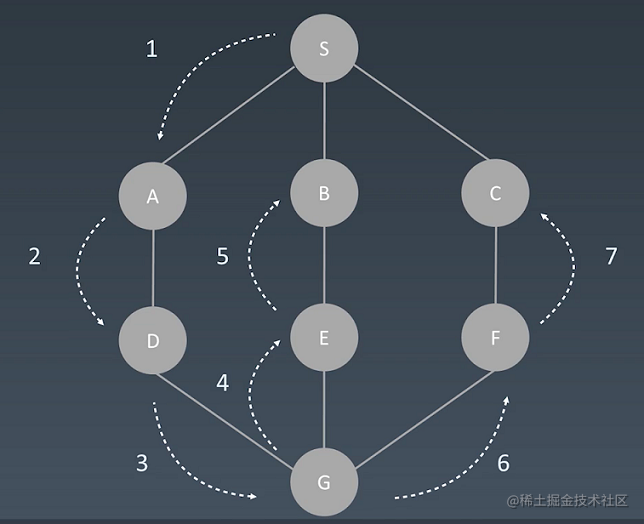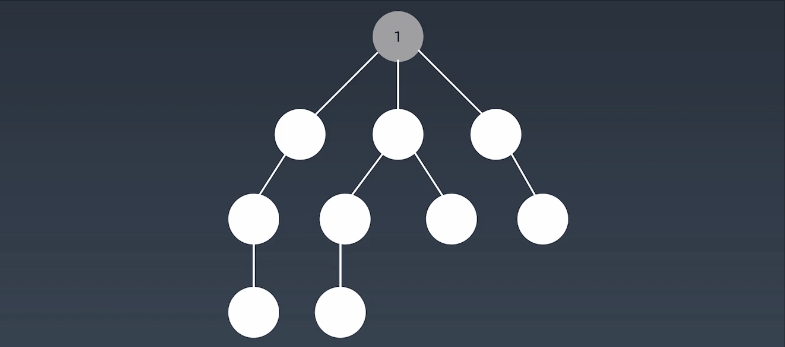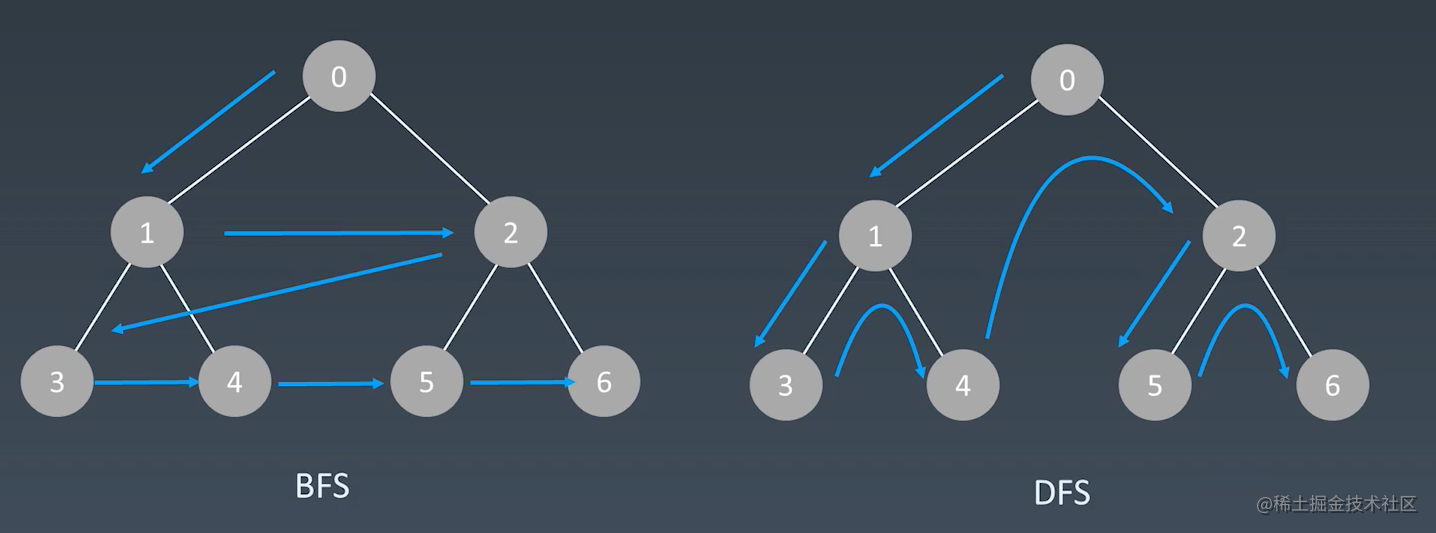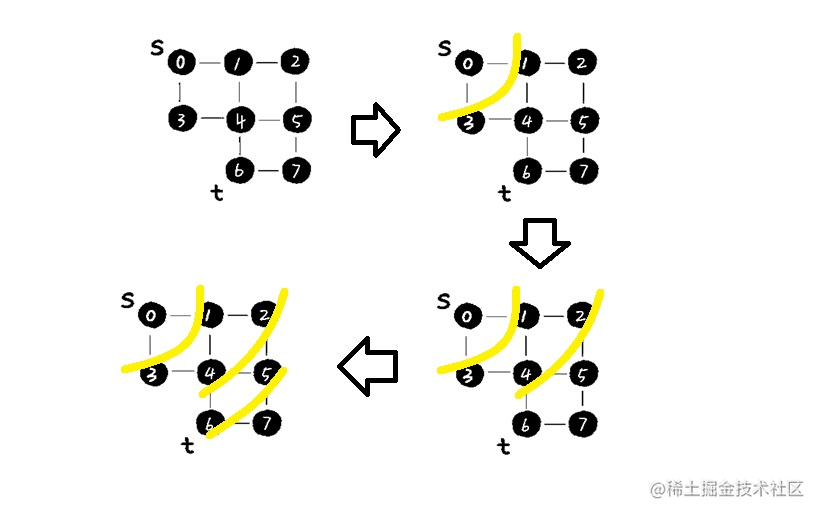# 迷人的两度搜索

## 1、BFS和DFS

### 1.1、深度优先搜索算法

1：实际上，回溯算法思想就是借助于深度优先搜索来实现的。

DFS负责搜索所有的路径，回溯辅以选择和撤销选择这种思想寻找可能的解，当然代码写起来基于递归（所以代码写起来就是用递归实现的）。

2：DFS跟回溯有什么关系呢？

DFS代码参考模板：

``````//Java
private void dfs(TreeNode root,int level,List<List<Integer>> results){
//terminal 已下探到最底部节点
if(results.size()==level){ // or root == null or node alread visited
return；
}
// process current level node here.

//drill down   if node not visited
if(root.left!=null){
dfs(root.left,level+1,results);
}
if(root.right!=null){
dfs(root.right,level+1,results);
}
}

``````//Java
public void dfs(Node node,List<Integer> res) {
//terminal
if (node == null) {
return;
}
//process current level logic
//drill down
List<Node> children = node.children;
for (Node n:children) {
// if node not visited  then dfs node
if (not visited) { // 在基于图的dfs中一般需要判断顶点是否已访问过
dfs(n,res);
}
}
}### 1.2、广度优先搜索算法dfs和bfs搜索方式的比较：BFS代码的参考模板：需要借助一个队列Queue（或Deque）

``````//Java
public class TreeNode {
int val;
TreeNode left;
TreeNode right;

TreeNode(int x) {
val = x;
}
}

public List<List<Integer>> levelOrder(TreeNode root) {
List<List<Integer>> allResults = new ArrayList<>();
if (root == null) {
return allResults;
}
//将根节点入队列
while (!nodes.isEmpty()) {
//每次循环开始时：队列中的元素的个数其实就是当前这一层节点的个数
int size = nodes.size();
List<Integer> results = new ArrayList<>();
for (int i = 0; i < size; i++) {
//从队列中取出每一个节点（取出这一层的每个节点）
TreeNode node = nodes.poll();
//将该节点的左右子节点入队列
if (node.left != null) {
}
if (node.right != null) {
}
}
}
return allResults;
}1：BFS适合在树或图中求解最近，最短等相关问题

2：DFS适合在树或图中求解最远，最深等相关问题

3：实际应用中基于图的应用居多

## 2、面试实战

### 102. 二叉树的层序遍历

``````class Solution {
public List<List<Integer>> levelOrder(TreeNode root) {
//特殊判断
if (root == null) {
return new ArrayList();
}
queue.offer(root);
List<List<Integer>> res = new ArrayList();
while (!queue.isEmpty()) {
int size = queue.size();
List<Integer> list = new ArrayList();
for (int i=0;i<size;i++) {
TreeNode poll = queue.poll();

//将左右子树节点加入队列
if (poll.left != null) {
queue.offer(poll.left);
}
if (poll.right != null) {
queue.offer(poll.right);
}
}
}
return res;
}
}

``````class Solution {
public List<List<Integer>> levelOrder(TreeNode root) {
List<List<Integer>> res = new ArrayList();
dfs(root,0,res);
return res;
}

public void dfs(TreeNode root,int level,List<List<Integer>> res) {
//terminal
if (root==null) {
return;
}

//将当前层的集合初始化好
int size = res.size();
if ( level > size-1) {
}
//将当前节点加到当前层对应的集合中
List<Integer> list = res.get(level);

//drill down
dfs(root.left,level+1,res);
dfs(root.right,level+1,res);

}
}

### 104. 二叉树的最大深度

``````max(l,r)+1

``````class Solution {
public int maxDepth(TreeNode root) {
return dfs(root);
}
//求一棵子树的最大深度
public int dfs(TreeNode node) {
//终止条件
if (node == null) {
return 0;
}
//左子树最大深度
int leftDepth = dfs(node.left);
//右子树最大深度
int rightDepth = dfs(node.right);
//当前节点的最大深度为左子树最大深度和右子树最大深度中的最大值+1
return Math.max(leftDepth,rightDepth) +1;
}
}

``````class Solution {
public int maxDepth(TreeNode root) {
//特殊判断
if (root==null) {
return 0;
}
int deep = 0;
while (!queue.isEmpty()) {
int size  = queue.size();
for (int i=0;i<size;i++) {
TreeNode p = queue.poll();
if (p.left!=null) {
queue.offer(p.left);
}
if (p.right!=null) {
queue.offer(p.right);
}
}
//每一层节点遍历完成后计数器+1
deep++;
}
return deep;
}
}

### 515. 在每个树行中找最大值

``````class Solution {
public List<Integer> largestValues(TreeNode root) {
List<Integer> res = new ArrayList();
if (root==null) {
return res;
}
queue.offer(root);
while (!queue.isEmpty()) {
int size = queue.size();

int max = Integer.MIN_VALUE;
for (int i=0;i<size;i++) {
TreeNode p = queue.poll();
if (p.left !=null) {
queue.offer(p.left);
}
if (p.right!=null) {
queue.offer(p.right);
}
//比较判断每一层的最大值
max = Math.max(max,p.val);
}
//保存每一层的最大值
}

return res;
}
}

### 200. 岛屿数量

``````class Solution {

//定义顶点向：上下左右，各走一步的方向信息
int[][] directions = {{0,1},{0,-1},{-1,0},{1,0}};

//定义网格的行数
int rows;
//定义网格的列数
int clos;

public int numIslands(char[][] grid) {
//定义岛屿总数
int count = 0;
//获取网格有多少行
rows = grid.length;
if (rows==0) {
return count;
}
//获取网格有多少列
clos = grid.length;
//定义网格各顶点是否被访问过
boolean[][] visited = new boolean[rows][clos];

//从图中去找能构成岛屿的顶点
for (int i=0;i<rows;i++) {
for (int j=0;j<clos;j++) {
//如果是陆地，并且没有被访问过则深度优先搜索(i,j)顶点相连的陆地。
if (grid[i][j] == '1' && !visited[i][j]) {
dfs(i,j,visited,grid);
//找到一块count+1
count++;
}
}
}
return count;

}
//搜索与(x,y)相连的陆地顶点，并标记这些顶点。
public void dfs(int x,int y,boolean[][] visited,char[][] grid) {
//走过的顶点要标记
visited[x][y] = true;
//从该顶点，向上下左右四个方向去走
for (int i=0;i< 4;i++) {
int newX = x + directions[i]; // directions[i]分别代表上下左右四个方向 directions[i]是X轴坐标要走的距离
int newY = y + directions[i];

//如果新顶点在网格内,且是陆地，且没有访问过,则继续搜索下去
if (inGrid(newX,newY) && grid[newX][newY]=='1' && !visited[newX][newY]) {
dfs(newX,newY,visited,grid);
}
}
}

//检查顶点(x,y)是否在网格内
public boolean inGrid(int x,int y) {
return x>=0 && x< rows && y>=0 && y<clos;
}
}

127. 单词接龙

529. 扫雷游戏

36. 有效的数独